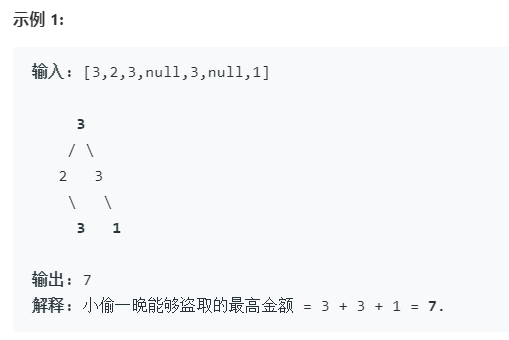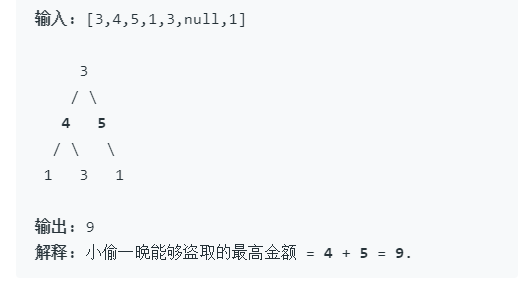# 打家劫舍III``````/**
* Definition for a binary tree node.
* struct TreeNode {
*     int val;
*     TreeNode *left;
*     TreeNode *right;
*     TreeNode(int x) : val(x), left(NULL), right(NULL) {}
* };
*/
class Solution {
public:
int rob(TreeNode* root) {
if(root == NULL){
return 0;
}
int money = root->val;
if(root->left){
money += rob(root->left->right) + rob(root->left->left);
}
if(root->right){
money += rob(root->right->left) + rob(root->right->right);
}
return max(money,rob(root->left)+rob(root->right));
}

};
``````